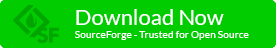# Increase the points that need to win the game

What is up buddy? In this article, we will continue to edit our pygame project by increasing the difficulty to win this game. 1) We will increase the points that we need to win the game by double. 2) We will also increase the damage points to 3 instead of 1 when the player gets hit by the missile from the horizontal moving enemy ship. We only need to update one file to make those changes, the overlap class.

We will come back and do more editions on this file later on but for now, let us edit the above-mentioned items first.

```from pygame.locals import *

class Overlap(object):

def __init__(self):
pass # nothing here

# is player and enemy, player missile, enemy missile overlap
def isOverlap(self, player, em, ex, score, gm):

self.checkOverlap(em.enemy_list, player, ex, gm, score, em.width, em.height, em.enemy_missile_manager.width, em.enemy_missile_manager.height, None)

if(gm.level_manager.get_level() == 3):
self.checkOverlap(em.horizontal_enemy_list, player, ex, gm, score, em.width1, em.height1, em.enemy_missile_manager.width, em.enemy_missile_manager.height, gm.level_manager.get_level())

def checkOverlap(self, e_list, player, ex, gm, score, width, height, m_width, m_height, level):

self.player_rect = Rect(player.pos.x, player.pos.y, player.width, player.height)

for i in range(len(e_list)):  # is player collides with enemy

self.em_rect = Rect(e_list[i].x, e_list[i].y, width, height)
if (self.player_rect.colliderect(self.em_rect)):
e_list[i].on = False
if (e_list[i].hit == False):
ex.create_explosion(player.pos.x + 2, player.pos.y + 2)
e_list[i].hit = True
gm.state = gm.OVER
gm.setup(gm.level_manager.get_level())

for i in range(len(e_list)):  # is enemy missile hits player

for j in range(len(e_list[i].missile_list)):
self.em_rect = Rect(e_list[i].missile_list[j].x, e_list[i].missile_list[j].y,
m_width, m_height)
if (self.player_rect.colliderect(self.em_rect)):
e_list[i].missile_list[j].on = False
ex.create_explosion(player.pos.x + 2, player.pos.y + 2)
if(level == 3):
score.set_score(-3)
else:
score.set_score(-1)
if (score.power_y &gt; 100):
gm.state = gm.OVER
gm.setup(gm.level_manager.get_level())

for i in range(len(e_list)):  # is player missile hits enemy

self.em_rect = Rect(e_list[i].x, e_list[i].y, width, height)

for j in range(len(player.getMissileManager().missile_list)):

self.mm_rect = Rect(player.getMissileManager().missile_list[j].x,
player.getMissileManager().missile_list[j].y, player.getMissileManager().width,
player.getMissileManager().height)

if (self.em_rect.colliderect(self.mm_rect)):

if (e_list[i].hit == False):
ex.create_explosion(e_list[i].x, e_list[i].y + 2)
e_list[i].hit = True
e_list[i].on = False
player.getMissileManager().missile_list[j].on = False

if(level == 3):
score.set_score(2)
else:
score.set_score(1)
if (score.score &gt;= gm.level_manager.get_level() * 60):
gm.level_manager.increase_level()

```

The changes above will not be shown in the latest version of this game immediately because I will only update this project once a week. By the way, have you downloaded this game already, if not then you can do so from the below widgets.Tomorrow I will start to write about the next project as well as continue with the present project, so stay tuned.